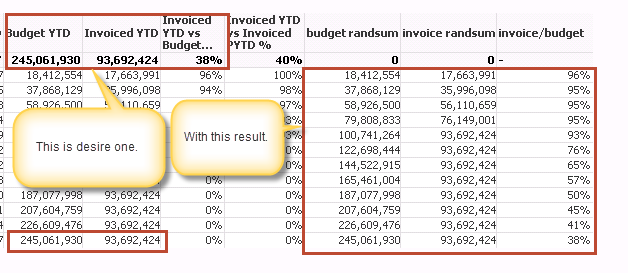# QlikView App Dev

Discussion Board for collaboration related to QlikView App Development.

Announcements
Support Case Portal has moved to Qlik Community! Read the FAQs to start exploring Support resources.
cancel
Showing results for
Did you mean:Creator II

## "Invoice YTD vs Budget YTD %" showing wrong result.

Hello all,

i am showing "invoice YTD" , "Budget YTD" and "Invoice YTD vs Budget YTD %" along with other column in one table. but "Invoice YTD vs Budget YTD %" is not showing data as per expectation.

formula for "Invoice YTD vs Budget YTD %":-

("invoice YTD"/"Budget YTD")*100

i have tried with Column(8)/column(7) formula in qlik view. also i have also tried by replacing formula for particular column with column() function but still it is showing wrong data.

my YTD formula areas per below:-

Budget YTD:-   =sum({<Common_Month = ,[Common_Day (#)]=,REC_TYPE = {'BudgetRec'}>}DAILY_BUDGET*XRATE_CONST*RATE)

with "Full Accumulation" property.

Invoice YTD:-

=Sum({<Common_Month = ,

REC_TYPE={InvoiceDateRec}>} (RAGLDA_ACCTD_AMOUNT*XRATE_CONST*RATE))

+

Sum({<Common_Month = ,

REC_TYPE = {'OfflineData'}>}ACCTD_AMOUNT*XRATE_CONST*RATE)

with "Full Accumulation" property.

1 Solution

Accepted SolutionsMVP

Try this:

RangeSum(Above(Sum({<Common_Month = , [Common_Day (#)] = , REC_TYPE = {'BudgetRec'}>} DAILY_BUDGET * XRATE_CONST * RATE), 0, RowNo()))

/

RangeSum(Above(Sum({<Common_Month = ,

REC_TYPE = {InvoiceDateRec}>} (RAGLDA_ACCTD_AMOUNT * XRATE_CONST * RATE))

+

Sum({<Common_Month = ,

REC_TYPE = {'OfflineData'}>} ACCTD_AMOUNT * XRATE_CONST * RATE), 0, RowNo()))

6 RepliesMVP

Try this:

RangeSum(Above(Sum({<Common_Month = , [Common_Day (#)] = , REC_TYPE = {'BudgetRec'}>} DAILY_BUDGET * XRATE_CONST * RATE), 0, RowNo()))

/

RangeSum(Above(Sum({<Common_Month = ,

REC_TYPE = {InvoiceDateRec}>} (RAGLDA_ACCTD_AMOUNT * XRATE_CONST * RATE))

+

Sum({<Common_Month = ,

REC_TYPE = {'OfflineData'}>} ACCTD_AMOUNT * XRATE_CONST * RATE), 0, RowNo()))Creator II
Author

it working exactly what i want only one problem i am facing and that Grand total, i am not able to get grand total for that particular column in which i have used RangSum().

Please take a look at screenshot.MVP

What would you expect to see there? Sum of accumulation or just the final accumulated number? I would think the later, but will wait for your response before I propose a solutionCreator II
Author

Hello,

for Budget YTD and Invoice YTD i need final accumulated number but for "Invoice YTD vs Budget YTD %" i need average.

Please find below Screen.MVP

For Budget and Invoice continue to use what you are using today (since it is working). For the percentage, you can set total mode to average on the expression tab of the straight table chart propertiesCreator II
Author

Thanks it working. 🙂Community Browser